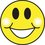# Set Theory Proof of $m^n < 2^{mn}$ for $m,n \in Z^{+}$

$n^m<2^{mn}$ for $m,n \in Z^{+}$

I chanced upon a rather interesting proof of this while studying sets, relations and functions in math class. Try to see if you can find it!

To get you started, consider two non-empty sets $A$ and $B$ containing $m$ and $n$ elements respectively. I'll post the solution in a few days...

SOLUTION

1. Consider two non-empty sets $A$ and $B$ containing $m$ and $n$ elements respectively.

2. The CARTESIAN PRODUCT of $A$ and $B$, given by $A \times B = \{(a,b):a \in A, b \in B\}$, is the set that contains all possible ordered pairs of the form $(a,b)$. Obviously, this set contains $mn$ elements.

3. A RELATION between two sets $A$ and $B$ is defined as a subset of their Cartesian product. Then, how many relations are possible between $A$ and $B$? This is the number of possible subsets of the Cartesian product, or, in other words, the number of elements in the POWER SET of $A \times B$. How do we find the number of possible subsets of $A \times B$? To choose a subset from a set, what do we do? We either choose an element to be part of the subset or not, i.e. we make a choice between 2 alternatives. Since there are $mn$ elements in the set, and we need to make a choice for each element, the number of subsets possible is given by $\boxed{2^{mn}}$.

4. A FUNCTION between two sets $A$ and $B$ is a subset of their Cartesian product too (just like a relation) but with an additional constraint: all elements of $A$ should have exactly one image in $B$. Then, how many functions are possible between $A$ and $B$? Since each element in $A$ has $n$ possible choices of an image in $B$ and there are $m$ elements in $A$, there are a total of $\boxed{n^{m}}$ possible functions from $A$ to $B$.

5. The final step is just logic: if a function is a relation with a constraint, there have to be fewer possible functions than relations.

Hence, $n^m < 2^{mn}$.Note by Raj Magesh
7 years ago

This discussion board is a place to discuss our Daily Challenges and the math and science related to those challenges. Explanations are more than just a solution — they should explain the steps and thinking strategies that you used to obtain the solution. Comments should further the discussion of math and science.

When posting on Brilliant:

• Use the emojis to react to an explanation, whether you're congratulating a job well done , or just really confused .
• Ask specific questions about the challenge or the steps in somebody's explanation. Well-posed questions can add a lot to the discussion, but posting "I don't understand!" doesn't help anyone.
• Try to contribute something new to the discussion, whether it is an extension, generalization or other idea related to the challenge.

MarkdownAppears as
*italics* or _italics_ italics
**bold** or __bold__ bold
- bulleted- list
• bulleted
• list
1. numbered2. list
1. numbered
2. list
Note: you must add a full line of space before and after lists for them to show up correctly
paragraph 1paragraph 2

paragraph 1

paragraph 2

[example link](https://brilliant.org)example link
> This is a quote
This is a quote
    # I indented these lines
# 4 spaces, and now they show
# up as a code block.

print "hello world"
# I indented these lines
# 4 spaces, and now they show
# up as a code block.

print "hello world"
MathAppears as
Remember to wrap math in $$ ... $$ or $ ... $ to ensure proper formatting.
2 \times 3 $2 \times 3$
2^{34} $2^{34}$
a_{i-1} $a_{i-1}$
\frac{2}{3} $\frac{2}{3}$
\sqrt{2} $\sqrt{2}$
\sum_{i=1}^3 $\sum_{i=1}^3$
\sin \theta $\sin \theta$
\boxed{123} $\boxed{123}$

Sort by:

${ m }^{ n }\quad ?{ \quad 2 }^{ mn }\\ { m }^{ n }\quad ?\quad { ({ 2 }^{ m }) }^{ n }\\ m\quad <\quad { 2 }^{ m }\\ { m }^{ n }\quad <{ \quad 2 }^{ mn }$

*I use the ? to show that the relation is not yet known

- 7 years ago

Well, of course you're right algebraically, but that's not the curious proof I saw. I'll post the solution soon, maybe in two more days... :)

- 7 years ago